Original post

The goal of this post is to show the of backpropagating a derivative for a
fully-connected (FC) neural network layer consisting of matrix multiplication
and bias addition. I have briefly mentioned this in an earlier post dedicated
to Softmax
,
but here I want to give some more attention to FC layers specifically.

Here is a fully-connected layer for input vectors with N elements, producing
output vectors with T elements: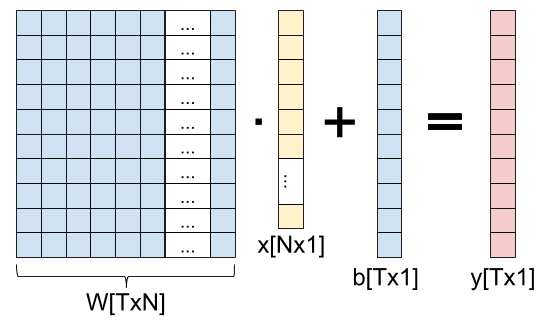As a formula, we can write:

[y=Wx+b]

Presumably, this layer is part of a network that ends up computing some loss
L. We’ll assume we already have the derivative of the loss w.r.t. the output
of the layer frac{partial{L}}{partial{y}}.

We’ll be interested in two other derivatives:
frac{partial{L}}{partial{W}} and
frac{partial{L}}{partial{b}}.

## Jacobians and the chain rule

As a reminder from The Chain Rule of Calculus,
we’re dealing with functions that map from n dimensions to m dimensions: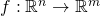. We’ll consider the outputs of f
to be numbered from 1 to m as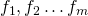. For each such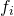we can compute its partial derivative by any of the n inputs as: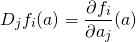Where j goes from 1 to n and a is a vector with n components. If f
is differentiable at a then the derivative of f at a is the Jacobian
matrix
: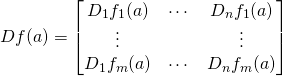The multivariate chain rule states: given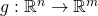and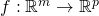and a point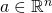,
if g is differentiable at a and f is differentiable at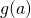then
the composition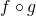is differentiable at a and its derivative
is: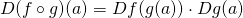Which is the matrix multiplication of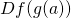and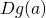.

## Back to the fully-connected layer

Circling back to our fully-connected layer, we have the loss L(y) – a
scalar function L:mathbb{R}^{T} to mathbb{R}. We also have the
function y=Wx+b. If we’re interested in the derivative w.r.t the
weights, what are the dimensions of this function? Our “variable part” is then
W, which has NT elements overall, and the output has T elements, so
y:mathbb{R}^{NT} to mathbb{R}^{T} .

The chain rule tells us how to compute the derivative of L w.r.t. W:

[frac{partial{L}}{partial{W}}=D(L circ y)(W)=DL(y(W)) cdot Dy(W)]

Since we’re backpropagating, we already know DL(y(W)); because of the
dimensionality of the L function, the dimensions of DL(y(W)) are
[1,T] (one row, T columns). y(W) has NT inputs and T outputs,
so the dimensions of Dy(W) are [T,NT]. Overall, the dimensions of
D(L circ y)(W) are then [1,NT]. This makes sense if you think about it,
because as a function of W, the loss has NT inputs and a single scalar
output.

What remains is to compute Dy(W), the Jacobian of y w.r.t. W. As
mentioned above, it has T rows – one for each output element of y, and NT
columns – one for each element in the weight matrix W. Computing such a large
Jacobian may seem daunting, but we’ll soon see that it’s very easy to generalize

[y_1=sum_{j=1}^{N}W_{1,j}x_{j}+b_1]

What’s the derivative of this result element w.r.t. each element in W? When
the element is in row 1, the derivative is x_j (j being the column
of W); when the element is in any other row, the derivative is 0.

Similarly for y_2, we’ll have non-zero derivatives only for the second
row of W (with the same result of x_j being the derivative for the
j-th column), and so on.

Generalizing from the example, if we split the index of W to i and j, we
get:

[begin{align}
D_{ij}y_t&=frac{partial(sum_{j=1}^{N}W_{t,j}x_{j}+b_t)}{partial W_{ij}}
&= left{begin{matrix}
x_j & i = t
0 & i ne t
end{matrix}right.
end{align*}]

This goes into row t, column (i-1)N+j in the Jacobian matrix. Overall,
we get the following Jacobian matrix with shape [T,NT]:

[Dy=begin{bmatrix}
x_1 & x_2 & cdots & x_N & cdots & 0 & 0 & cdots & 0
vdots & ddots & ddots & ddots & ddots & ddots & ddots & ddots & vdots
0 & 0 & cdots & 0 & cdots & x_1 & x_2 & cdots & x_N
end{bmatrix}]

Now we’re ready to finally multiply the Jacobians together to complete the
chain rule:

[D(L circ y)(W)=DL(y(W)) cdot Dy(W)]

The left-hand side is this row vector:

[DL(y)=(frac{partial L}{partial y_1}, frac{partial L}{partial y_2},cdots,frac{partial L}{partial y_T})]

And we’re multiplying it by the matrix Dy shown above. Each item in the
result vector will be a dot product between DL(y) and the corresponding
column in the matrix Dy. Since Dy has a single non-zero element
in each column, the result is fairly trivial. The first N entries are:

[frac{partial L}{partial y_1}x_1,
frac{partial L}{partial y_1}x_2,
cdots,
frac{partial L}{partial y_1}x_N]

The next N entries are:

[frac{partial L}{partial y_2}x_1,
frac{partial L}{partial y_2}x_2,
cdots,
frac{partial L}{partial y_2}x_N]

And so on, until the last (T-th) set of N entries is all x-es multiplied
by frac{partial L}{partial y_T}.

To better see how to apply each derivative to a corresponding element in W, we
can “re-roll” this result back into a matrix of shape [T,N]:

[frac{partial{L}}{partial{W}}=D(Lcirc y)(W)=begin{bmatrix}
frac{partial L}{partial y_1}x_1 & frac{partial L}{partial y_1}x_2 & cdots & frac{partial L}{partial y_1}x_N
frac{partial L}{partial y_2}x_1 & frac{partial L}{partial y_2}x_2 & cdots & frac{partial L}{partial y_2}x_N
vdots & vdots & ddots & vdots
frac{partial L}{partial y_T}x_1 & frac{partial L}{partial y_T}x_2 & cdots & frac{partial L}{partial y_T}x_N
end{bmatrix}]

## Computational cost and shortcut

While the derivation shown above is complete and mathematically correct, it can
also be computationally intensive; in realistic scenarios, the full Jacobian
matrix can be really large. For example, let’s say our input is a (modestly
sized) 128×128 image, so N=16,384. Let’s also say that T=100. The weight
matrix then has NT=1,638,400 elements; respectably big, but nothing out of the
ordinary.

Now consider the size of the full Jacobian matrix: it’s T by NT, or over
160 million elements. At 4 bytes per element that’s more than half a GiB!

Moreover, to compute every backpropagation we’d be forced to multiply this full
Jacobian matrix by a 100-dimensional vector, performing 160 million
multiply-and-add operations for the dot products. That’s a lot of compute.

But the final result D(Lcirc y)(W) is the size of W – 1.6 million
elements. Do we really need 160 million computations to get to it? No, because
the Jacobian is very sparse – most of it is zeros. And in fact, when we look
at the D(Lcirc y)(W) found above – it’s fairly straightforward to
compute using a single multiplication per element.

Moreover, if we stare at the frac{partial{L}}{partial{W}} matrix a
bit, we’ll notice it has a familiar pattern: this is just the outer product between the vectors
frac{partial{L}}{partial{y}} and x:

[frac{partial L}{partial W}=frac{partial L}{partial y}otimes x]

If we have to compute this backpropagation in Python/Numpy, we’ll likely write
code similar to:

```# Assuming dy (gradient of loss w.r.t. y) and x are column vectors, by
# performing a dot product between dy (column) and x.T (row) we get the
# outer product.
dW = np.dot(dy, x.T)
```

We’ve just seen how to compute weight gradients for a fully-connected layer.
Computing the gradients for the bias vector is very similar, and a bit simpler.

This is the chain rule equation applied to the bias vector:

[frac{partial{L}}{partial{b}}=D(L circ y)(b)=DL(y(b)) cdot Dy(b)]

The shapes involved here are: DL(y(b)) is still [1,T], because the
number of elements in y remains T. Dy(b) has T inputs (bias
elements) and T outputs (y elements), so its shape is [T,T]. Therefore, the
shape of the gradient D(L circ y)(b) is [1,T].

To see how we’d fill the Jacobian matrix Dy(b), let’s go back to the
formula for y:

[y_1=sum_{j=1}^{N}W_{1,j}x_{j}+b_1]

When derived by anything other than b_1, this would be 0; when derived
by b_1 the result is 1. The same applies to every other element of y:

[frac{partial y_i}{partial b_j}=left{begin{matrix}
1 & i=j
0 & ineq j
end{matrix}right]

In matrix form, this is just an identity matrix with dimensions [T,T].
Therefore:

[frac{partial{L}}{partial{b}}=D(L circ y)(b)=DL(y(b)) cdot I =DL(y(b))]

For a given element of b, its gradient is just the corresponding element in
frac{partial L}{partial y}.

## Fully-connected layer for a batch of inputs

The derivation shown above applies to a FC layer with a single input vector x
and a single output vector y. When we train models, we almost always try to
do so in batches (or mini-batches) to better leverage the parallelism of
modern hardware. So a more typical layer computation would be:

[Y=WX+b]

Where the shape of X is [N,B]; B is the batch size, typically a
not-too-large power of 2, like 32. W and b still have the same shapes, so
the shape of Y is [T,B]. Each column in X is a new input vector (for a
total of B vectors in a batch); a corresponding column in Y is the output.

As before, given frac{partial{L}}{partial{Y}}, our goal is to find
frac{partial{L}}{partial{W}} and
frac{partial{L}}{partial{b}}. While the end results are fairly simple
and pretty much what you’d expect, I still want to go through the full Jacobian
computation to show how to find the gradiends in a rigorous way.

Starting with the weigths, the chain rule is:

[frac{partial{L}}{partial{W}}=D(L circ Y)(W)=DL(Y(W)) cdot DY(W)]

The dimensions are:

• DL(Y(W)): [1,TB] because Y has T outputs for each input vector in
the batch.
• DY(W): [TB,TN] since Y(W) has TB outputs and TN
inputs overall.
• D(Lcirc Y)(W): [1,TN] same as in the batch-1 case, because the same
weight matrix is used for all inputs in the batch.

Also, we’ll use the notation x_{i}^{[b]} to talk about the i-th
element in the b-th input vector x (out of a total of B such input
vectors).

With this in hand, let’s see how the Jacobians look; starting with
DL(Y(W)), it’s the same as before except that we have to take the batch
into account. Each batch element is independent of the others in loss
computations, so we’ll have:

[frac{partial L}{partial y_{i}^{[b]}}]

As the Jacobian element; how do we arrange them in a 1-dimensional vector with
shape [1,TB]? We’ll just have to agree on a linearization here – same as we did
with W before. We’ll go for row-major again, so in 1-D the array Y would be:

[Y=(y_{1}^{},y_{1}^{},cdots,y_{1}^{[B]},
y_{2}^{},y_{2}^{},cdots,y_{2}^{[B]},cdots)]

And so on for T elements. Therefore, the Jacobian of L w.r.t Y is:

[frac{partial L}{partial Y}=(
frac{partial L}{partial y_{1}^{}},
frac{partial L}{partial y_{1}^{}},cdots,
frac{partial L}{partial y_{1}^{[B]}},
frac{partial L}{partial y_{2}^{}},
frac{partial L}{partial y_{2}^{}},cdots,
frac{partial L}{partial y_{2}^{[B]}},cdots)]

To find DY(W), let’s first see how to compute Y. The i-th element
of Y for batch b is:

[y_{i}^{[b]}=sum_{j=1}^{N}W_{i,j}x_{j}^{[b]}+b_i]

Recall that the Jacobian DY(W) now has shape [TB,TN]. Previously we had
to unroll the [T,N] of the weight matrix into the rows. Now we’ll also have to
unrill the [T,B] of the output into the columns. As before, first all b-s for
t=1, then all b-s for t=2, etc. If we carefully compute the derivative,
we’ll see that the Jacobian matrix has similar structure to the single-batch
case, just with each line repeated B times for each of the batch elements:

[DY(W)=begin{bmatrix}
x_{1}^{} & x_{2}^{} & cdots & x_{N}^{} & cdots & 0 & 0 & cdots & 0
x_{1}^{} & x_{2}^{} & cdots & x_{N}^{} & cdots & 0 & 0 & cdots & 0
vdots & ddots & ddots & ddots & ddots & ddots & ddots & ddots & vdots
x_{1}^{[B]} & x_{2}^{[B]} & cdots & x_{N}^{[B]} & cdots & 0 & 0 & cdots & 0
vdots & ddots & ddots & ddots & ddots & ddots & ddots & ddots & vdots
0 & 0 & cdots & 0 & cdots & x_{1}^{} & x_{2}^{} & cdots & x_{N}^{}
0 & 0 & cdots & 0 & cdots & x_{1}^{} & x_{2}^{} & cdots & x_{N}^{}
vdots & ddots & ddots & ddots & ddots & ddots & ddots & ddots & vdots
0 & 0 & cdots & 0 & cdots & x_{1}^{[B]} & x_{2}^{[B]} & cdots & x_{N}^{[B]}
end{bmatrix}]

Multiplying the two Jacobians together we get the full gradient of L w.r.t.
each element in the weight matrix. Where previously (in the non-batch case) we

[frac{partial L}{partial W_{ij}}=frac{partial L}{partial y_i}x_j]

[frac{partial L}{partial W_{ij}}=sum_{b=1}^{B}frac{partial L}{partial y_{i}^{[b]}}x_{j}^{[b]}]

Which makes total sense, since it’s simply taking the loss gradient computed
from each batch separately and adds them up. This aligns with our intuition of
how gradient for a whole batch is computed – compute the gradient for each batch

As before, there’s a clever way to express the final gradient using matrix
operations. Note the sum across all batch elements when computing
frac{partial L}{partial W_{ij}}. We can express this as the matrix
multiplication:

[frac{partial L}{partial W}=frac{partial L}{partial Y}cdot X^T]

This is a good place to recall the computation cost again. Previously we’ve seen
that for a single-input case, the Jacobian can be extremely large ([T,NT] having
about 160 million elements). In the batch case, the Jacobian would be even
larger since its shape is [TB,NT]; with a reasonable batch of 32, it’s something
like 5-billion elements strong. It’s good that we don’t actually have to hold
the full Jacobian in memory and have a shortcut way of computing the gradient.

## Bias gradient for a batch

For the bias, we have:

[frac{partial{L}}{partial{b}}=D(L circ Y)(b)=DL(Y(b)) cdot DY(b)]

DL(Y(b)) here has the shape [1,TB]; DY(b) has the shape [TB,T].
Therefore, the shape of frac{partial{L}}{partial{b}} is [1,T], as
before.

From the formula for computing Y:

[y_1=sum_{j=1}^{N}W_{1,j}x_{j}+b_1]

We get, for any batch b:

[frac{partial y_{i}^{[b]}}{partial b_j}=left{begin{matrix}
1 & i=j
0 & ineq j
end{matrix}right]

So, whereas DY(b) was an identity matrix in the no-batch case, here it
looks like this:

[DY(b)=begin{bmatrix}
1 & 0 & 0 & cdots & 0
1 & 0 & 0 & cdots & 0
vdots & vdots & vdots & ddots & vdots
1 & 0 & 0 & cdots & 0
0 & 1 & 0 & cdots & 0
0 & 1 & 0 & cdots & 0
vdots & vdots & vdots & ddots & vdots
0 & 0 & 0 & cdots & 1
0 & 0 & 0 & cdots & 1
vdots & vdots & vdots & ddots & vdots
0 & 0 & 0 & cdots & 1
end{bmatrix}]

With B identical rows at a time, for a total of TB rows. Since
frac{partial L}{partial Y} is the same as before, their matrix
multiplication result has this in column j:

[frac{partial{L}}{partial{b_j}}=sum_{b=1}^{B}frac{partial L}{partial y_{j}^{[b]}}]

Which just means adding up the gradient effects from every batch element
independently.

This post started by explaining that the parameters of a fully-connected layer
we’re usually looking to optimize are the weight matrix and bias. In most cases
this is true; however, in some other cases we’re actually interested in
propagating a gradient through x – often when there are more layers before
the fully-connected layer in question.

Let’s find the derivative frac{partial{L}}{partial{x}}. The chain
rule here is:

[frac{partial{L}}{partial{x}}=D(L circ y)(x)=DL(y(x)) cdot Dy(x)]

Dimensions: DL(y(x)) is [1, T] as before; Dy(x) has T outputs
(elements of y) and N inputs (elements of x), so its dimensions are [T, N].
Therefore, the dimensions of frac{partial{L}}{partial{x}} are [1, N].

From:

[y_1=sum_{j=1}^{N}W_{1,j}x_{j}+b_1]

We know that frac{partial y_1}{partial x_j}=W_{1,j}. Generalizing
this, we get frac{partial y_i}{partial x_j}=W_{i,j}; in other words,
Dy(x) is just the weight matrix W. So
frac{partial{L}}{partial{x_i}} is the dot product of DL(y(x))
with the i-th column of W.

Computationally, we can express this as follows:

```dx = np.dot(dy.T, W).T
```

Again, recall that our vectors are column vectors. Therefore, to multiply dy
from the left by W we have to transpose it to a row vector first. The result
of this matrix multiplication is a [1, N] row-vector, so we transpose it again
to get a column.

An alternative method to compute this would transpose W rather than dy and
then swap the order:

```dx = np.dot(W.T, dy)
```

These two methods produce exactly the same dx; it’s important to be familiar
with these tricks, because otherwise it may be confusing to see a transposed W
when we expect the actual W from gradient computations.

  As explained in the softmax post, we linearize the 2D matrix W into a single vector with NT elements using some approach like row-major, where the N elements of the first row go first, then the N elements of the second row, and so on until we have NT elements for all the rows. This is a fully general approach as we can linearize any-dimensional arrays. To work with Jacobians, we’re interested in K inputs, no matter where they came from – they could be a linearization of a 4D array. As long as we remember which element out of the K corresponds to which original element, we’ll be fine.
  In some cases you may hear about averaging the gradients across the batch. Averaging just means dividing the sum by B; it’s a constant factor that can be consolidated into the learning rate.